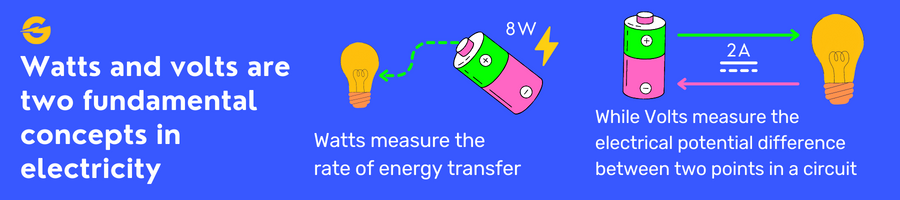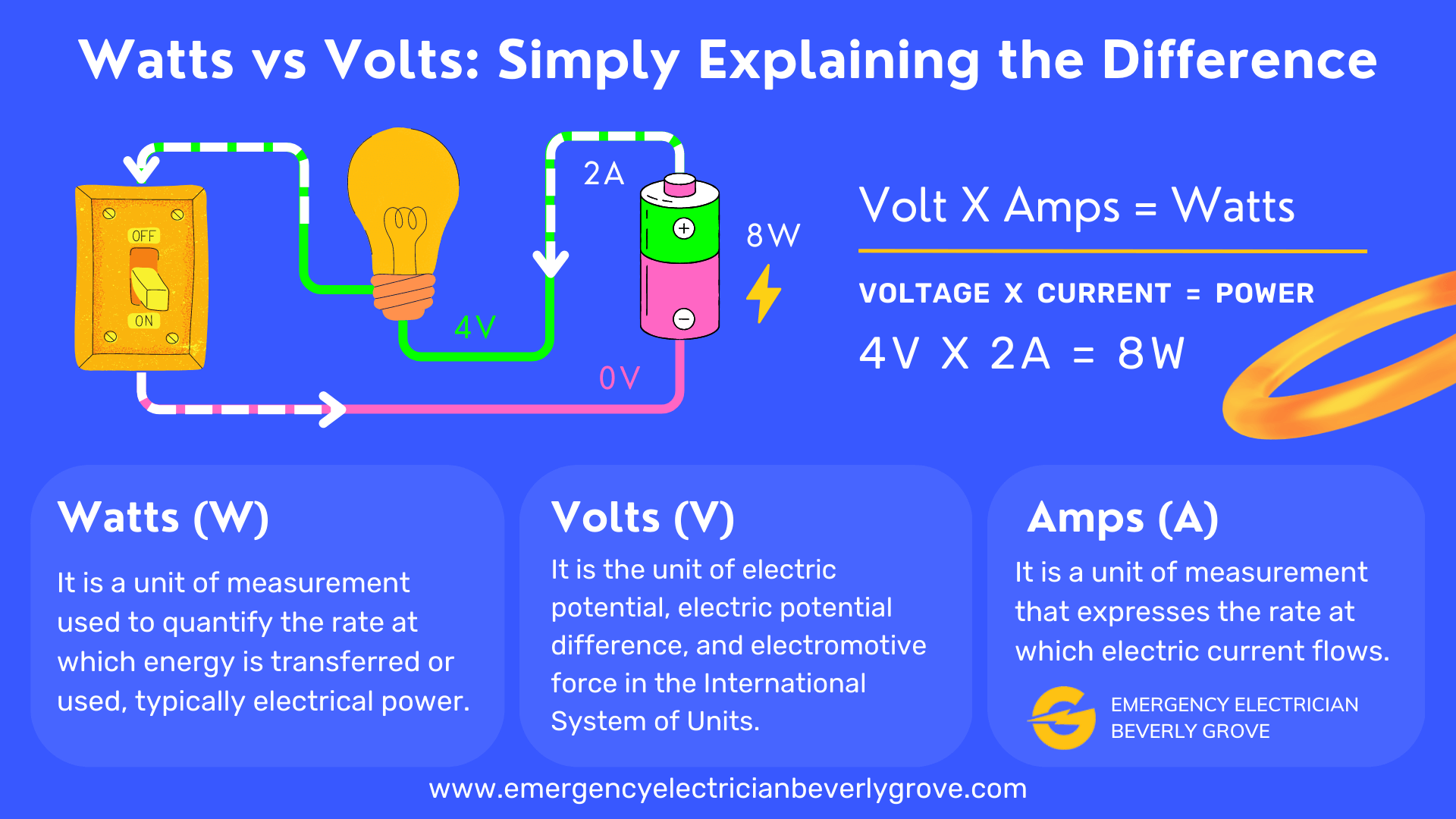# Key Differences between Watts and Volts: A Comprehensive Guide

## What Is a Watt?

A watt (W) is the unit of power used to describe the rate of energy conversion or transfer in electrical systems. Named after the Scottish inventor James Watt, this unit quantifies the work done by electrical devices or circuits over time. In the context of electricity, a watt represents the amount of energy an electrical device consumes or generates per unit of time. One watt is equal to one joule of energy transferred or converted per second.

## What Is a Volt and Voltage?

A volt (V) is the unit of electric potential, also known as voltage. It measures the potential energy per unit charge in an electrical system. The term “volt” is derived from the name of Alessandro Volta, an Italian scientist known for his pioneering work in electricity. Voltage can be thought of as the force that pushes electric current through a conductor. It influences the amount of current flowing through a circuit, with higher voltage levels corresponding to a greater flow of current.## What Is an Amp and Current?

An ampere (A), or simply “amp,” is the unit of electric current. It denotes the flow of electric charge through a conductor over a given period of time. In essence, current refers to the movement of electrons in a circuit. The flow of current depends on the voltage level and the resistance present in the circuit. According to Ohm’s Law, the current flowing through a circuit is directly proportional to the voltage and inversely proportional to the resistance.

## What are Ohms and Resistance?

An ohm (Ω) is the unit of electrical resistance, signifying the opposition to the flow of current in an electrical circuit. Resistance depends on factors such as the material of the conductor, its length, and its cross-sectional area. Ohm’s Law defines the relationship between voltage, current, and resistance as Voltage (V) = Current (I) * Resistance (R). This fundamental law helps us understand how these three variables interact in an electrical circuit.

## Watts vs. VoltsGrasping the differences between watts and volts is crucial for anyone working with electrical systems. The primary distinctions can be outlined as follows:

• Watts are the unit of power, while volts are the unit of electric potential.
• Watts measure the rate of energy transfer or conversion, whereas volts measure the force driving the flow of electric current.
• Watts indicate the energy consumption or generation of a device, while volts represent the potential energy within a circuit.

The relationship between watts, volts, and amps can be expressed using the formula: Power (P) = Voltage (V) * Current (I). This equation demonstrates the interconnected nature of power, voltage, and current in an electrical system.

### The Wattage of Different Home Appliances

The table below provides an overview of the approximate wattage of various home appliances. Understanding the wattage of appliances enables consumers to gauge their energy consumption and make well-informed decisions regarding electrical device usage.

Appliance Wattage
LED light bulb 8-10W
Refrigerator 150-400W
Microwave 600-1200W
Television 60-400W
Laptop 50-100W
Air conditioner 1000-5000W
Vacuum cleaner 500-1500W
Electric kettle 1000-1800W
Hair dryer 800-1800W
Washing machine 500-1500W
Toaster 800-1500W
Blender 300-1000W
Iron 1000-1800W
Electric oven 1000-5000W
Coffee maker 800-1400W
Dishwasher 1200-1500W
Ceiling fan 65-175W
Space heater 1000-1500W
Electric stove 1000-3000W
Desktop computer 400-800W
Gaming console 100-300W

## F.A.Q.

1. Are volts and watts the same?
No, volts and watts are not the same. Volts are the unit of electric potential or voltage, while watts are the unit of power.
2. Are there any relationships between watts and volts?
Yes, there is a relationship between watts and volts. The power (P) in watts is equal to the product of voltage (V) and current (I) in amperes. The formula is: P = V * I.
3. How to calculate Watts from Volts with formula?
To calculate watts from volts, you need to know the current (I) in amperes. Using the formula P = V * I, you can find the power (P) in watts by multiplying the voltage (V) in volts by the current (I) in amperes.
4. What are the instruments used to measure watts and volts?
Watts and volts can be measured using various instruments. A multimeter is a versatile device that can measure both voltage and current, from which watts can be calculated. Alternatively, a dedicated wattmeter can be used to measure power directly in watts. Voltmeters are specifically designed to measure voltage, while ammeters measure current.